# AQA A Level Chemistry复习笔记1.6.2 Energy Level Diagrams

### Energy Level Diagrams

• An energy level diagram is a diagram that shows the energies of the reactants, the transition state(s) and the products of the reaction as the reaction proceeds ( called 'extent of the reaction' below)
• The transition state is a stage during the reaction at which chemical bonds are partially broken and formed
• The transition state is very unstable – a molecule in the transition state cannot be isolated and is higher in energy than the reactants and products
• The activation energy (Ea) is the energy needed to reach the transition state
• We can define the activation energy as ‘the minimum amount of energy needed for reactant molecules to have a successful collision and start the reaction’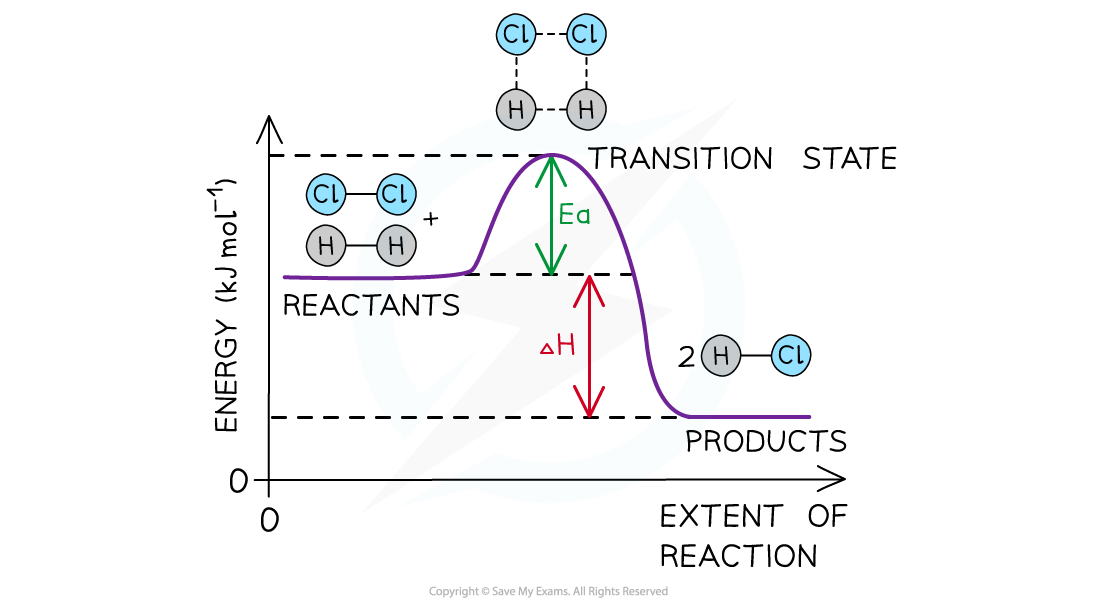The energy level diagram for the reaction of hydrogen with chlorine to form hydrogen chloride gas

#### Exothermic reaction

• In an exothermic reaction, the reactants are higher in energy than the products
• The reactants are therefore closer in energy to the transition state
• This means that exothermic reactions have a lower activation energy compared to endothermic reactions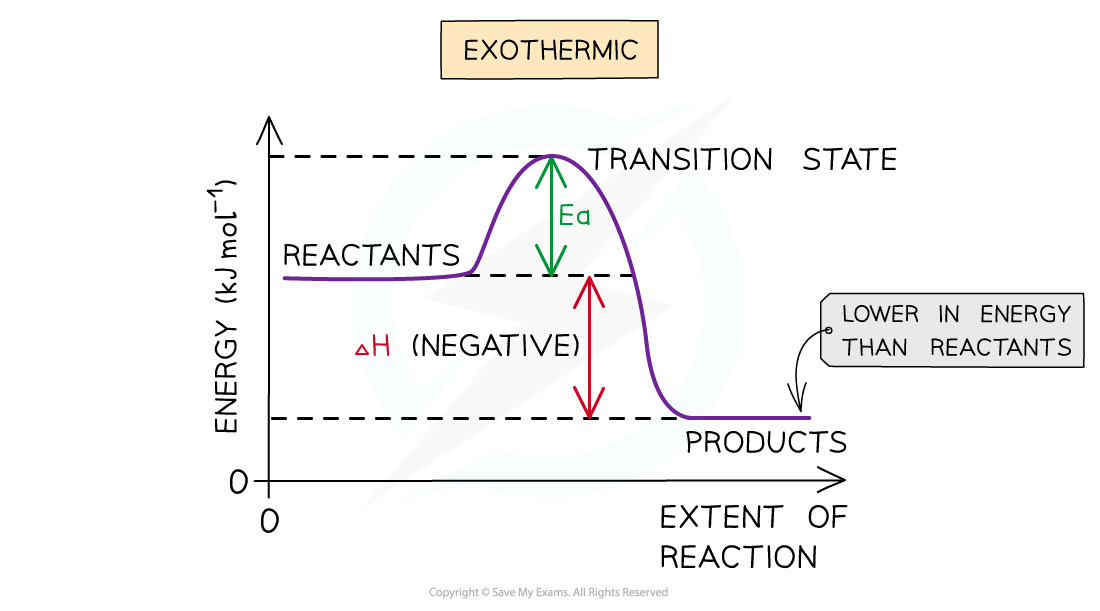The energy level diagram for exothermic reactions. ∆H is negative because the system (reactants) loses energy to the surroundings.

#### Endothermic reaction

• In an endothermic reaction, the reactants are lower in energy than the products
• The reactants are therefore further away in energy to the transition state
• This means that endothermic reactions have a higher activation energy compared to exothermic reactions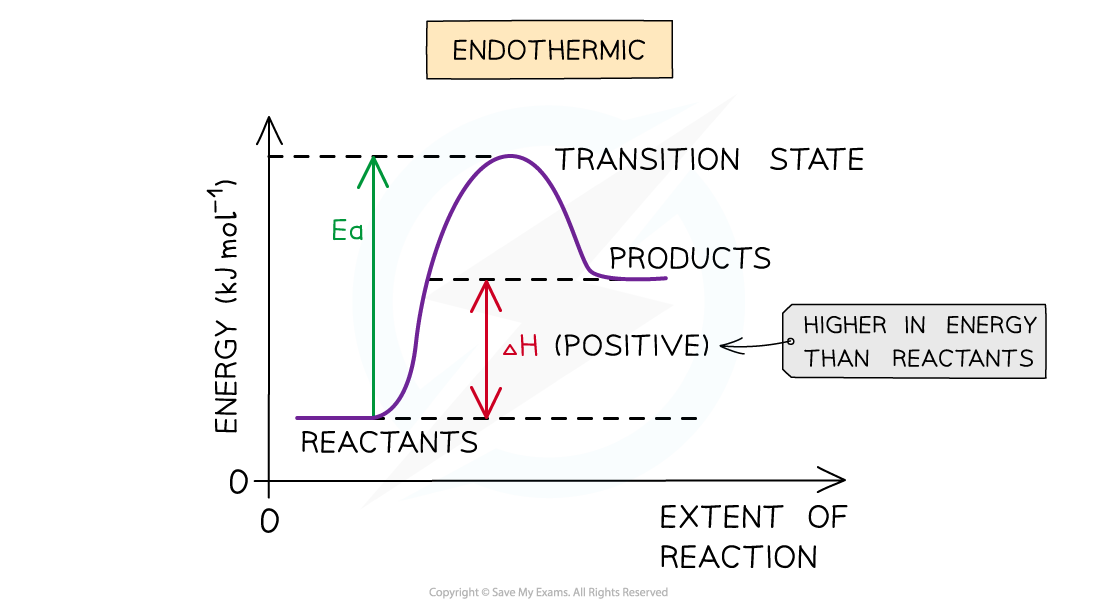The energy level diagram for endothermic reactions. The system (reactant substances) have gained energy so ∆H is positive.

#### Worked Example

Drawing energy level diagrams of the combustion of methaneThe Ea and ΔH for the complete combustion of methane are +2653 kJ mol-1 and -890 kJ mol-1 respectively. Draw the energy level diagram for this reaction.

Step 1: The chemical equation for the complete combustion of methane is:

CH4 (g) + 2O2 (g) → CO2 (g) + 2H2O (l)

Step 2: Combustion reactions are always exothermic (ΔH is negative) so the reactants should be drawn higher in energy than the products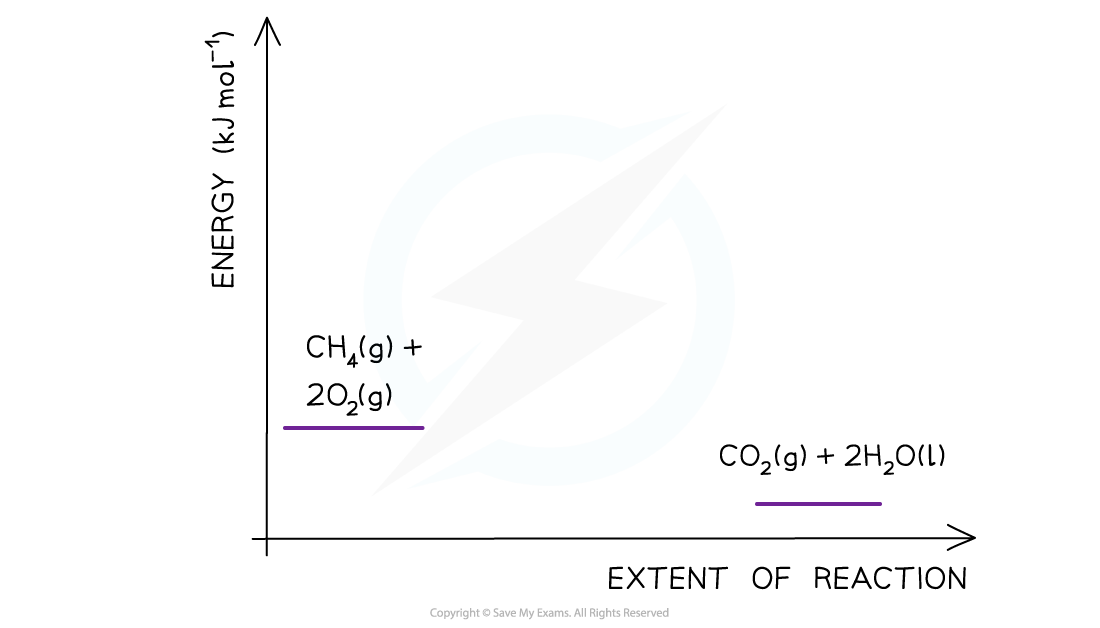Step 3: Draw the curve in the energy level diagram clearly showing the transition state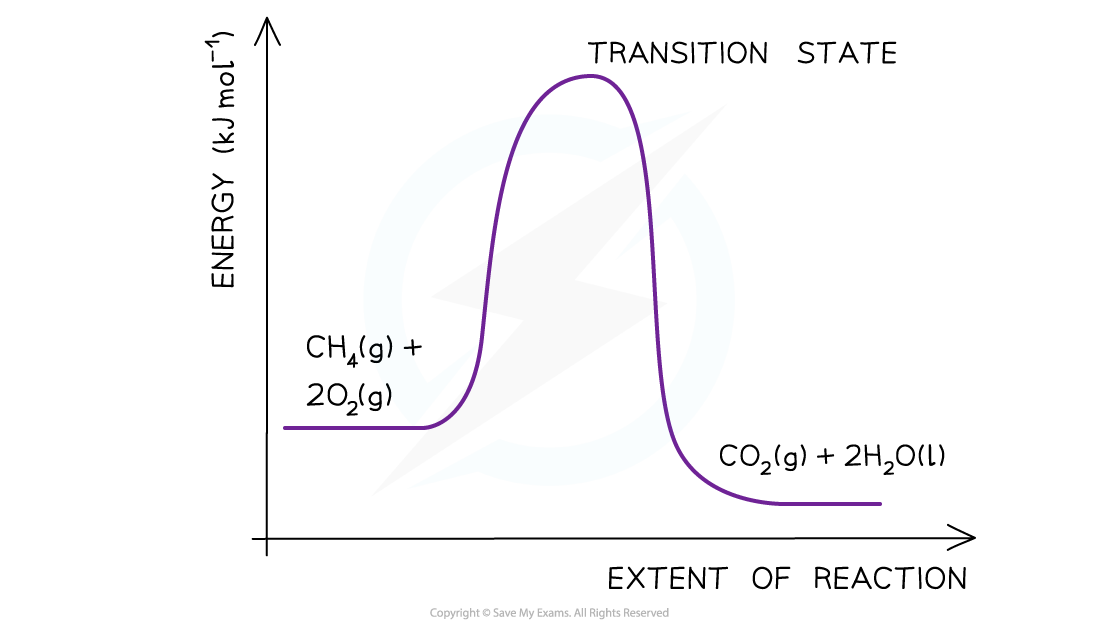Step 4: Draw arrows to show the Ea and ΔH  including their values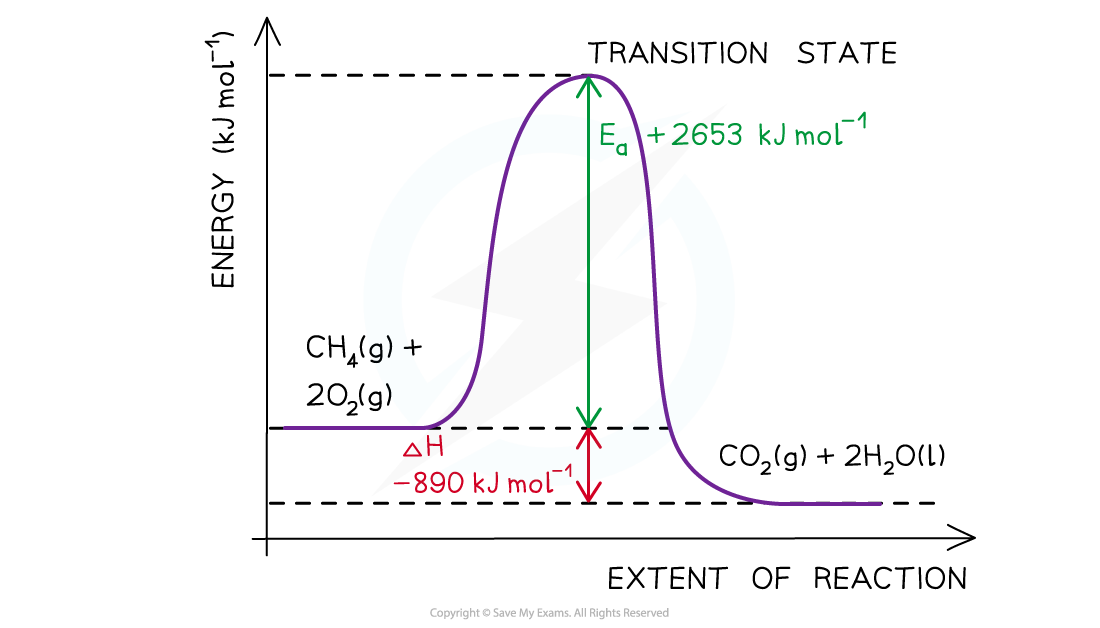#### Worked Example

Determining the activation energyΔH for a reaction is +70 kJ mol-1 and Ea for the reverse reaction is +20 kJmol-1. Use the reaction pathway diagram below to determine the Ea for the forward reaction.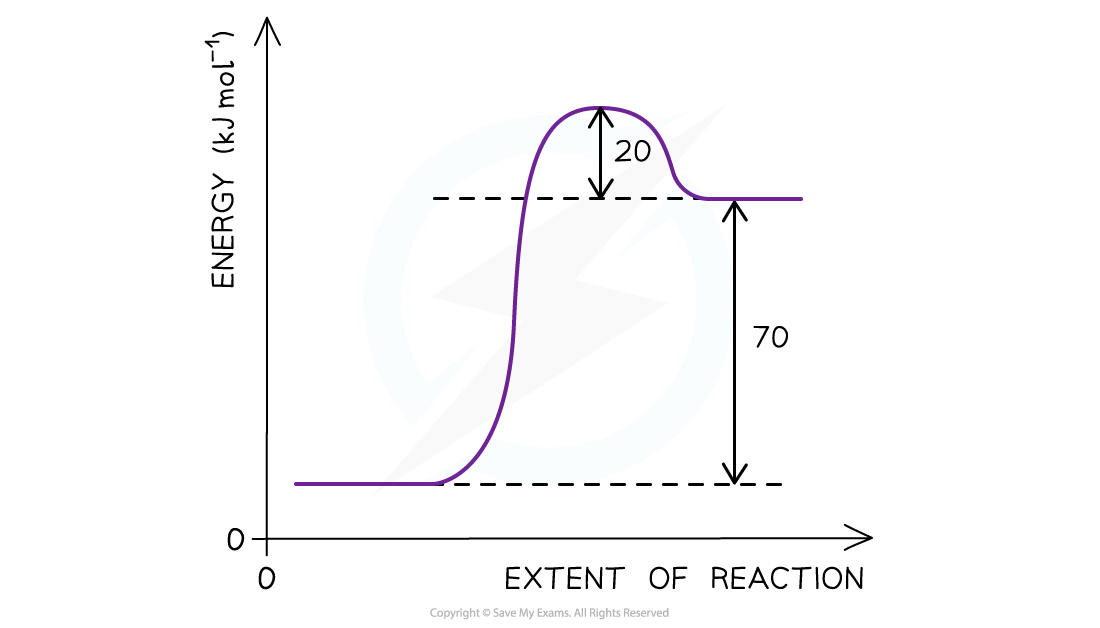The reaction pathway diagram for a reversible reaction

• The Ea  is the energy difference from the energy level of the reactants to the top of the ‘hump’
• Ea (forward reaction) = (+70 kJ mol-1) + (+ 20 kJ mol-1 ) = +90 kJ mol-1

#### Exam Tip

The activation energy is the energy difference from reactants to transition state.The enthalpy change of the reaction is the energy difference from reactants to products.Remember to label the axis of the energy level diagrams!